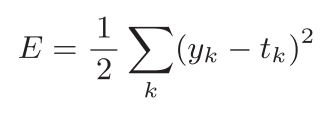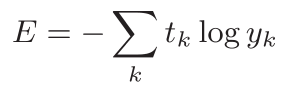# python深度学习第二讲——用python写深度学习损失函数

1.可以用作损失函数的有均方误差：def mean_squared_error(y, t):
return 0.5 * np.sum((y-t)**2)
>>> # 设“2”为正确解
>>> t = [0, 0, 1, 0, 0, 0, 0, 0, 0, 0]
>>>
>>> # 例1：“2”的概率最高的情况（0.6）
>>> y = [0.1, 0.05, 0.6, 0.0, 0.05, 0.1, 0.0, 0.1, 0.0, 0.0]
>>> mean_squared_error(np.array(y), np.array(t))
0.097500000000000031
>>>
>>> # 例2：“7”的概率最高的情况（0.6）
>>> y = [0.1, 0.05, 0.1, 0.0, 0.05, 0.1, 0.0, 0.6, 0.0, 0.0]
>>> mean_squared_error(np.array(y), np.array(t))
0.59750000000000003

2.除了均方误差之外，交叉熵误差（cross entropy error）也经常被用作损失函数。交叉熵误差如下式所示：def cross_entropy_error(y, t):
delta = 1e-7
return -np.sum(t * np.log(y + delta))

>>> t = [0, 0, 1, 0, 0, 0, 0, 0, 0, 0]
>>> y = [0.1, 0.05, 0.6, 0.0, 0.05, 0.1, 0.0, 0.1, 0.0, 0.0]
>>> cross_entropy_error(np.array(y), np.array(t))
0.51082545709933802
>>>
>>> y = [0.1, 0.05, 0.1, 0.0, 0.05, 0.1, 0.0, 0.6, 0.0, 0.0]
>>> cross_entropy_error(np.array(y), np.array(t))
2.3025840929945458©️2019 CSDN 皮肤主题: Age of Ai 设计师: meimeiellie